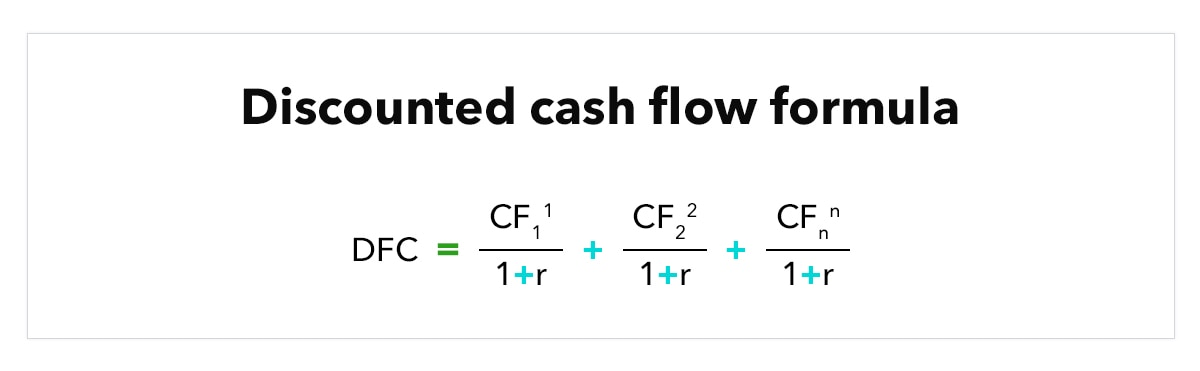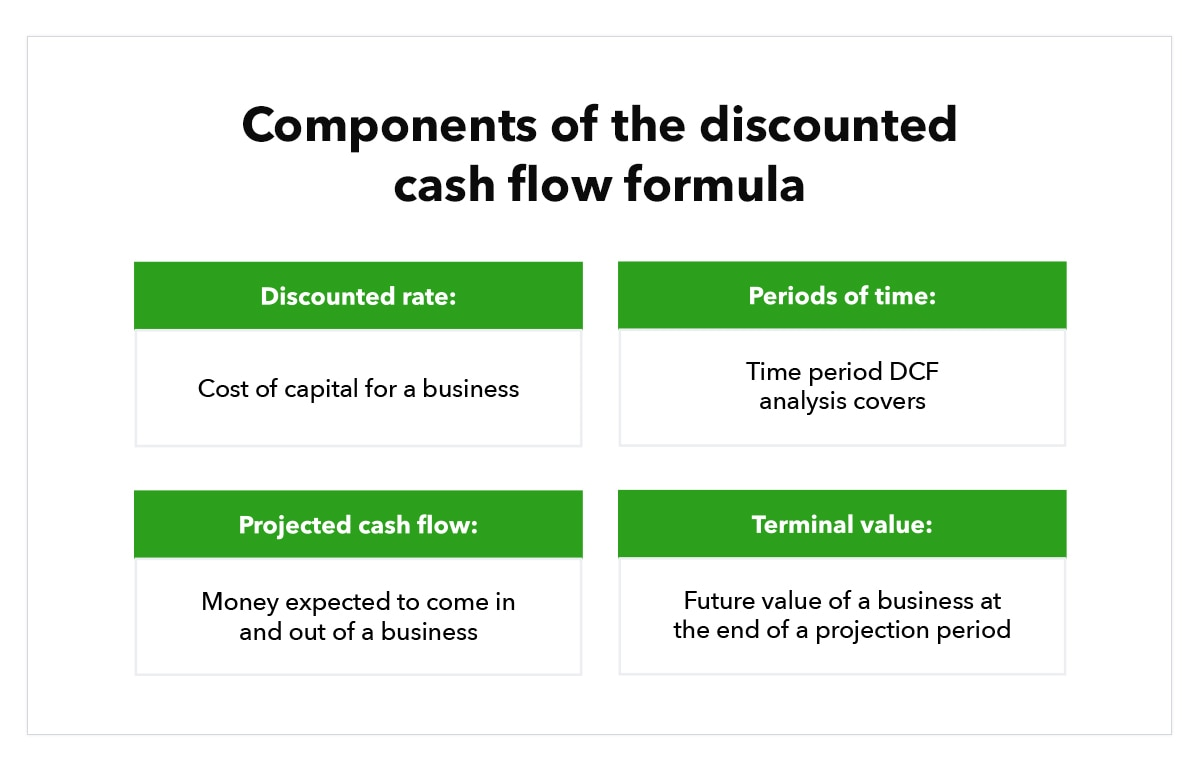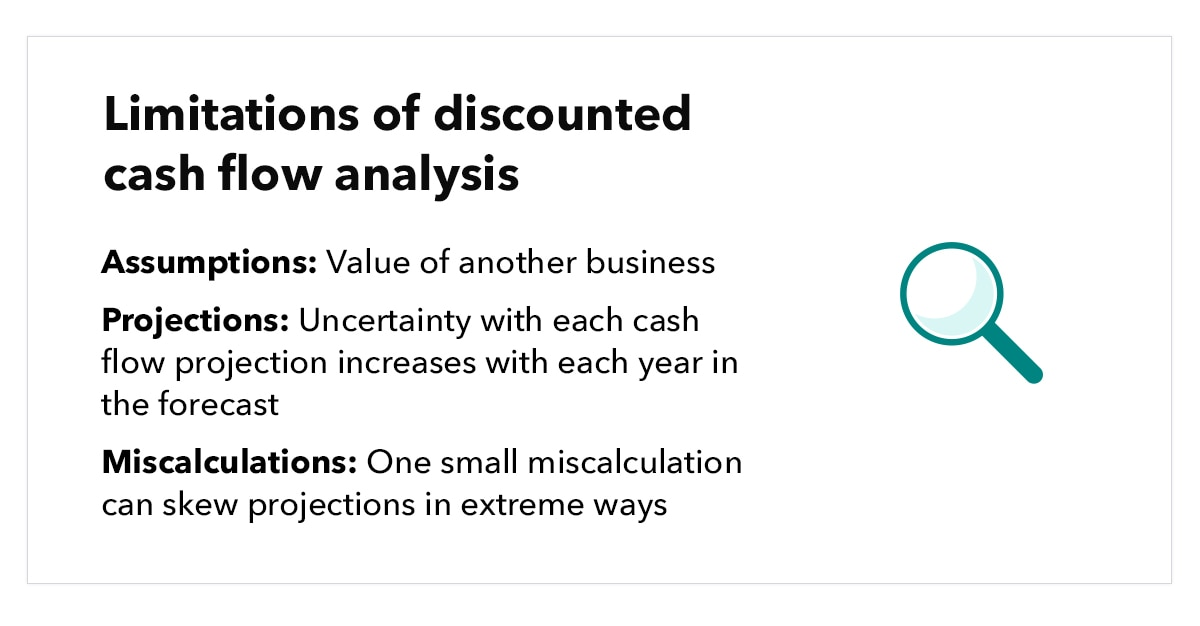How the discounted cash flow formula works

Photo from unsplash

Originally Posted On: How the discounted cash flow formula works | QuickBooks (intuit.com)

Whether you’re a business owner, working in corporate finance, or an investor, knowing how much an investment is worth is crucial. Many businesses have to figure out ways to continue growing their company. In most cases, this means investing in certain areas of the company, such as a new piece of equipment or a large project. But will these investments pay off? That’s where the discounted cash flow (DCF) analysis comes in.

Simply put, DCF can help you determine the value of an asset. This guide will go over the DCF calculation so you can make future cash flow projections, understand the value of a company, and more. Read through to learn how to put the DCF formula into practice, or use the list below to jump to a section of your choice.

What is discounted cash flow?

Cash flow represents how much money goes in and out of a business’s doors. There are several ways to measure cash flow, such as the free cash flow (FCF), cash flow from operating activities (CFO), and levered cash flow (LCF) methods. One cash flow measurement we’ll discuss in this article is the discounted cash flow method.

Discounted cash flow (DCF) is a valuation method that businesses use to estimate how much an asset is worth by using future cash flows. In other words, DCF analysis looks at how much money an investment will make over time. Businesses can then use it to compare to other investments to see which one will provide the biggest benefit. This calculation describes the time value of money. This is a concept that shows how cash available to a company today is worth more than the same amount in the future.

By knowing how much an investment is worth, business owners can decide whether to stick with it or walk away.

Why calculate discounted cash flow

Calculating discounted cash flow can be beneficial for many reasons. Some reasons why a business or investor will use the DCF formula include:

• Getting the value of an entire business
• Finding the value of a bond
• Determining the value of a company’s investment or project
• Calculating the value of shares in a company
• Finding the value of a business’s cost-saving project
• Getting the value of anything that produces or affects a company’s cash flow

There are many instances where a company might decide to use the DCF formula. However, it’s important to remember that DCF is based on many assumptions and might not always be accurate.

How to calculate discounted cash flow

Now that you know what DCF is and why it’s used, it’s time to look at the DCF formula. DCF is measured by dividing the expected yearly earnings by a discount rate that’s based on the weighted average cost of capital (WACC) by dispensing debt. The DCF formula is as follows:

DCF = (CF / (1+r)1) + (CF / (1+r)2) + (CF / (1+r)3) + (…) + (CF / (1+r)n)Components of the formula

To calculate DCF, we need to consider certain factors. The discounted cash flow analysis uses these elements to help predict how much an asset is worth today:

• Discount rate: In the discounted cash flow formula, “r” refers to the discount rate. The discount rate is the cost of capital for a business, which is similar to an interest rate on future cash inflows. It’s common to use the WACC, which is the rate a business pays to finance its assets.
• Periods of time: In the DCF formula, “n” refers to the period of time, which can be expressed as years, quarters, months, or any other time period. Many companies refer to the IRS’s depreciation guidelines to determine how much time to look at.
• Projected cash flow: In the DCF formula, “CF” refers to the projected cash flow for the given year. Projected cash flow is the amount of money that’s expected to come in and out of your business during a specific period of time.
• Terminal value: The terminal value measures the future value of a business at the end of the projection period in the DCF analysis. It’s used to forecast the perpetual growth rate, typically beyond five years in the future. This is because getting a reliable estimate of how a business will perform that far in advance can be difficult.With the DCF model, a company discounts its projected cash flow using an annual rate using its WACC as its discount rate. Discounting the WACC gives businesses a net present value estimate (NPV), which can help them determine an investment’s potential.

Discounted cash flow formula example

Now that we know the DCF formula, let’s put it into practice with a hypothetical example. Let’s say you look at your business’s balance sheet. You find that the business brings in \$400,000 each year in free cash flows and has a steady rate of 5% each year.

To calculate your cash flow (CF), you’ll multiply \$400,000 by 0.05 to get 5% of \$400,000, which gives you \$20,000. You’ll then add \$20,000 to \$400,000 to get \$420,000, which is your cash flow (CF2) for the next year. To calculate CF3, you will then find 5% of \$420,000, which is \$441,000.

Depending on how far you want to forecast, you can continue repeating the cash flow calculation. For this example, however, we’ll stick to forecasting three years out. Now that your cash flow is calculated, you can begin calculating the value of the company with the DCF formula. So far, we have three calculations:

• CF1; \$400,000
• CF2: \$420,000
• CF3: \$441,000

Now, let’s say you have a target compounded rate of return of 15% per year, which will represent “r” in the DCF formula. Plug this into the DCF equation:

DCF = (\$400,000 / (1+0.15)1) + (\$420,000 / (1+0.15)2) + (\$441,000 / (1+0.15)3)

DCF = \$347,826 + \$317,580 + \$289,965

DCF = \$955,371

In our example, your business’s present value would be worth \$955,371 over a three-year period. The DCF equation translates future cash flows into how much they’re valued to you today. This is based on the compounded rate of return (15% in this example) you think you can achieve with your money today. In this case, the growth rate (15%) is higher than your company’s cash flow (5%). A higher growth rate means the discounted versions of your future cash flows will depreciate each year until they bottom out at zero.

Whether you’re buying real estate, shares of stock, or an entire business, or trying to find the required rate of return for a project, the DCF formula can help.

Limitations of discounted cash flow analysis

While the DCF analysis is said to be one of the more accurate valuation methods, it does have its drawbacks. Some limitations to the DCF analysis when valuing a business include:• Assumptions: The DCF method is based mostly on assumptions. Business owners can easily make unrealistic predictions, which can end up giving them an inflated projection of future cash flow. For example, the terminal rate for startups is often higher than that of established businesses. As for the discount rate, companies often choose the WACC, which is mostly theoretical and not always representative of real life.
• Projections: With the DCF model, cash flow forecasting can extend years and even decades, depending on how far in the future you want to predict. However, the uncertainty with each cash flow projection rises with each year in the forecast. The outermost years in the model can be a complete shot in the dark. With that said, most companies stick to five or 10 years’ worth of forecasting.
• Miscalculations: The DCF model is very sensitive—one small miscalculation can skew projections in extreme ways. As a business owner, it’s important to ensure that all calculations are accurate. Many use Microsoft Excel to help prevent calculating errors.